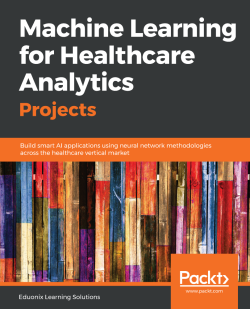# Machine Learning for Healthcare Analytics Projects5 (1 reviews total)
By Eduonix Learning Solutions
• \$20.99 eBook
• \$24.99 Print + eBook
• \$12.99 eBook + Subscription
What do you get with a Packt Subscription?

• Constantly updated with 100+ new titles each month
• Breadth and depth in over 1,000+ technologies

Machine Learning (ML) has changed the way organizations and individuals use data to improve the efficiency of a system. ML algorithms allow strategists to deal with a variety of structured, unstructured, and semi-structured data. Machine Learning for Healthcare Analytics Projects is packed with new approaches and methodologies for creating powerful solutions for healthcare analytics.

This book will teach you how to implement key machine learning algorithms and walk you through their use cases by employing a range of libraries from the Python ecosystem. You will build five end-to-end projects to evaluate the efficiency of Artificial Intelligence (AI) applications for carrying out simple-to-complex healthcare analytics tasks. With each project, you will gain new insights, which will then help you handle healthcare data efficiently. As you make your way through the book, you will use ML to detect cancer in a set of patients using support vector machines (SVMs) and k-Nearest neighbors (KNN) models. In the final chapters, you will create a deep neural network in Keras to predict the onset of diabetes in a huge dataset of patients. You will also learn how to predict heart diseases using neural networks.

By the end of this book, you will have learned how to address long-standing challenges, provide specialized solutions for how to deal with them, and carry out a range of cognitive tasks in the healthcare domain.

Publication date:
October 2018
Publisher
Packt
Pages
134
ISBN
9781789536591

# Breast Cancer Detection

Machine learning, a subset of artificial intelligence (AI), has taken the world by storm. Within the healthcare domain, it is possible to see how machine learning can make manual processes easier, providing benefits for patients, providers, and pharmaceutical companies alike. Google, for example, has developed a machine learning algorithm that can identify cancerous tumors on mammograms. Similarly, Stanford University has developed a deep learning algorithm to identify skin cancer.

In this chapter, we will discuss how one can use machine learning to detect breast cancer. We will look at the following topics:

• Objective of this project
• Detecting breast cancer with SVM and KNN
• Data visualization with machine learning
• Relationships between variables
• Understanding machine learning algorithms
• Training models
• Predictions in machine learning

# Objective of this project

The main objective of this chapter is to see how machine learning helps detect cancer through the SVM and KNN models. The following screenshot is an example of the final output that we are trying to achieve in this project: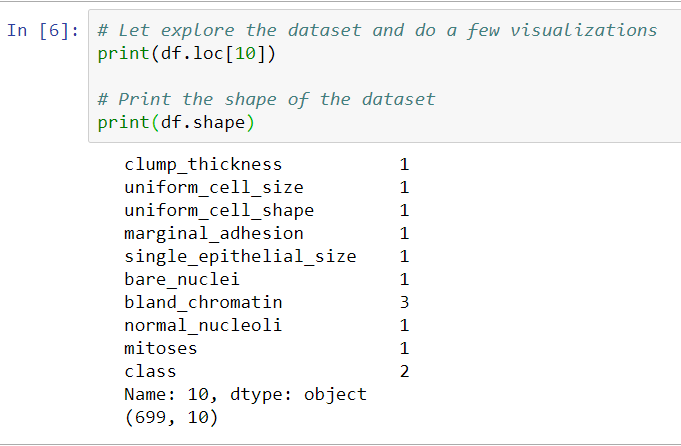We will receive the information shown in the preceding screenshot for approximately 700 cells in our dataset. This will include factors such as clump_thickness, marginal_adhesion, bare_nuclei, bland_chromatin, and mitoses, all of which are properties that would be valuable for a pathologist. In the screenshot, you can see that the class is 4, which means that it is malignant; so, this particular cell is cancerous. A class of 2, on the other hand, would be benign, or healthy.

Now, let's take a look at the models that we will be training as the chapter progresses in the following screenshot: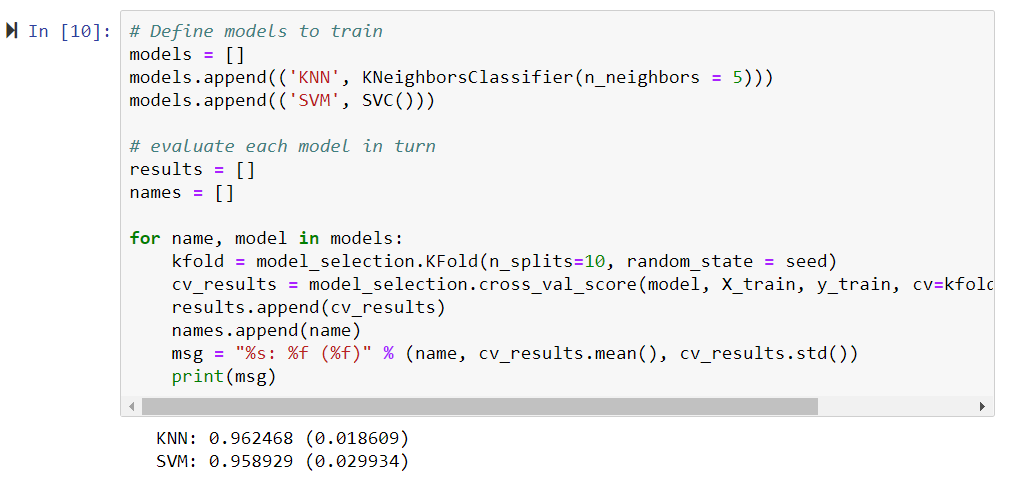Based on the cell's information, both models have predicted that the cell is cancerous, or malignant. In this project, we will go through the steps required to achieve this goal. We will start by downloading and installing packages with Anaconda, we will move on to starting a Jupyter Notebook, and then you will learn how to program these machine learning models in Python.

# Detecting breast cancer with SVM and KNN models

In this section, we will take a look at how to detect breast cancer with a support vector machine (SVM). We're also going to throw in a k-nearest neighbors (KNN) clustering algorithm, and compare the results. We will be using the conda distribution, which is a great way to download and install Python since conda is a package manager, meaning that it makes downloading and installing the necessary packages easy and straightforward. With conda, we're also going to install the Jupyter Notebook, which we will use to program in Python. This will make sharing code and collaborating across different platforms much easier.

Now, let's go through the steps required to use Anaconda, as follows:

2. Open up a Command Prompt, which is the best way to use conda, and go into the Tutorial folder.
3. If conda is in your Path variables, you can simply type conda install, followed by whichever package you need. We're going to be using numpy, so we will type that, as you can see in the following screenshot: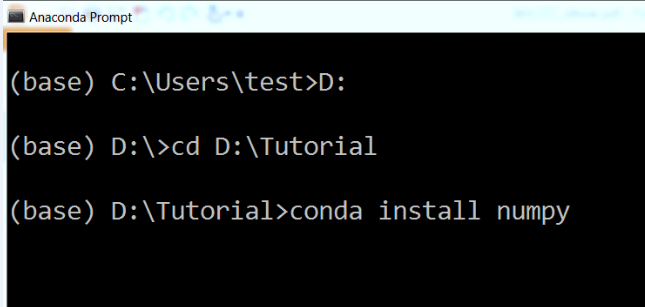If you get an error saying that the command conda was not found, it means that conda isn't in the Path variables. Edit the environment variables and add conda.
1. To start the Jupyter Notebook, simply type jupyter notebook and press Enter. If conda is in the path, Jupyter will be found, as well, because it's located in the same folder. It will start to load up, as shown in the following screenshot: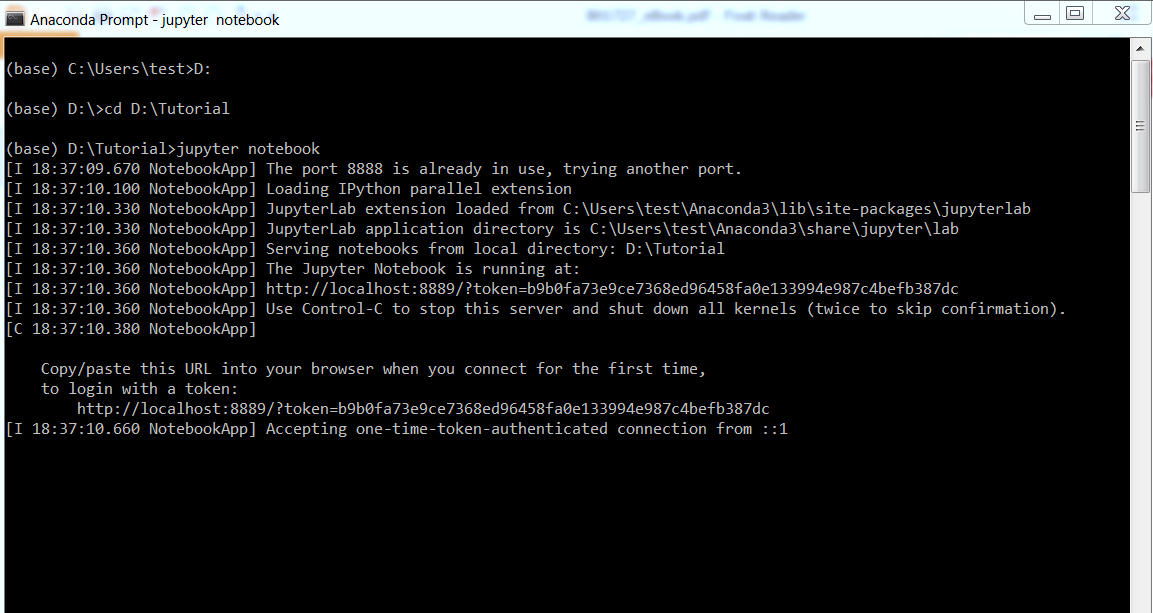The folder that we're in when we type jupyter notebook is where it will open up on the web browser.

1. After that, click on New, and select Python [default]. Using Python 2.7 would be preferable, as it seems to be more of a standard in the industry.
2. To check that we all have the same versions, we will conduct an import step.
3. Rename the notebook to Breast Cancer Detection with Machine Learning.
4. Import sys, so that we can check whether we're using Python 2.7.
We will need to import numpy for computational operations and arrays, matplotlib for plotting, pandas to handle the datasets, and sklearn, to get the machine learning packages.
1. We will import numpy, matplotlib, pandas, and the sklearn packages and print their versions. We can view the changes in the following screenshot: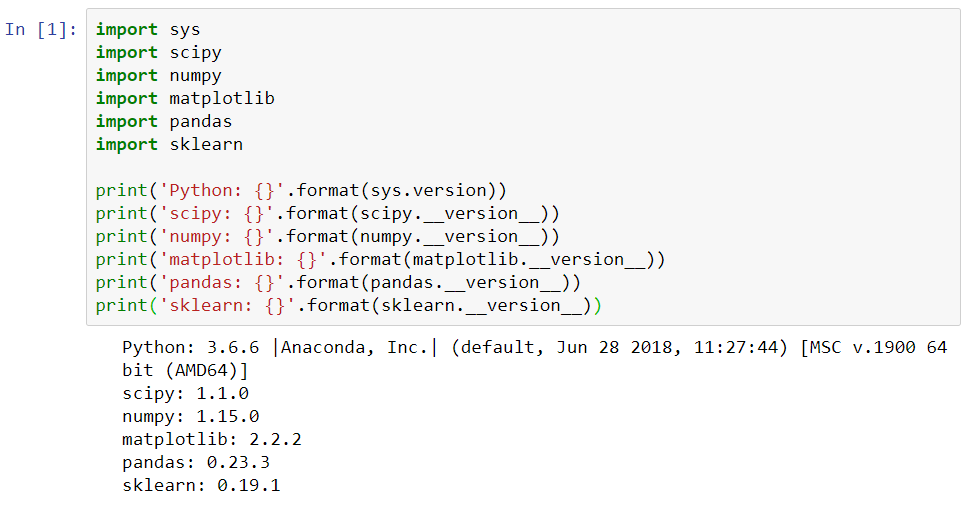To run the cell in Jupyter Notebook, simply press Shift + Enter. A number will pop up when it completes, and it'll print out the statements. Once again, if we encounter errors in this step and we are unable to import any of the preceding packages, we have to exit the Jupyter Notebook, type conda install, and mention whichever package we are missing in the Terminal. These will then be installed. The necessary packages and versions are shown as follows:

• Python 2.7
• 1.14 for NumPy
• Matplotlib
• Pandas
• Sklearn

The following screenshot illustrates how to import these libraries in the specific way that we're going to use them in this project: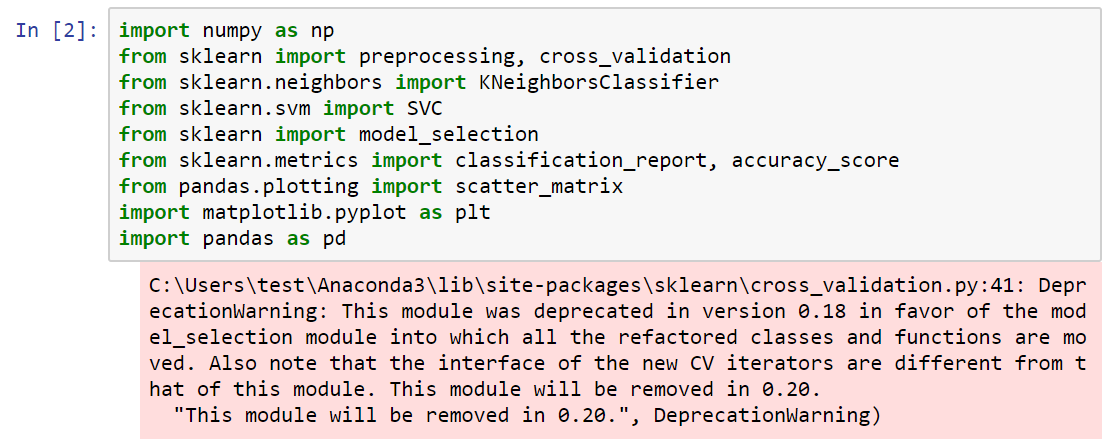In the following steps, we will look at how to import the different arguments in these libraries:

1. First, we will import NumPy, using the command import numpy as np.
2. Next, we will import the various classes and functions in sklearn - namely, preprocessing and cross_validation.
3. From neighbors, we will import KNeighborsClassifier, which will be KNN.
4. From sklearn.svm, we will import the support vector classifier (SVC).
5. We're going to do model_selection, so that we can use both KNN and SVC in one step.
6. We will then get some metrics, in which we will import the classification_report, as well as the accuracy_score.
7. From pandas, we need to import plotting, which is the scatter_matrix. This will be useful when we're exploring some data visualizations, before diving into the actual machine learning.
8. Finally, from matplotlib.pyplot, we will import pandas as pd.
9. Now, press Shift + Enter, and make sure that all of the arguments import.
You may get a deprecation warning, as shown in the preceding screenshot. That is because some of these packages are getting old.
1. Now that we have all of our packages set up, we can move on to loading the dataset. This is where we're going to be getting our information from. We're going to be using the UCI repository, since they have a large collection of datasets for machine learning, and they're free and available for everybody to use.
2. The URL that we're going to use can be imported directly, if we type the whole URL. This is going to import our dataset with 11 different columns. We can see the URL and the various columns in the following screenshot: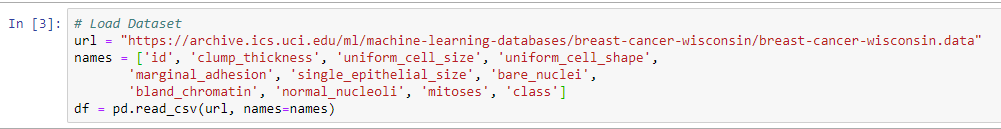We will then import the cell data. This will include the following aspects:

• The first column will simply be the ID of the cell
• In the second column, we will have clump_thickness
• In the third column, we will have uniform_cell_size
• In the fourth column, we will have uniform_cell_shape
• In the fifth column, we will have marginal_adhesion
• In the sixth column, we will have signle_epithelial_size
• In the seventh column, we will have bare_nuclei
• In the eighth column, we will have bland_chromatin
• In the ninth column, we will have normal_nucleoli
• In the tenth column, we will have mitoses
• And finally, in the eleventh column, we will have class

These are factors that a pathologist would consider to determine whether or not a cell had cancer. When we discuss machine learning in healthcare, it has to be a collaborative project between doctors and computer scientists. While a doctor can help by indicating which factors are important to include, a computer scientist can help by carrying out machine learning. Now, let's move on to the next steps:

1. Since we've got the names of our columns, we will now start a DataFrame.
2. The next step will be to add pd, which stands for pandas. We're going to use the function read_csv_url, which means that the names will be equal to those listed previously.
1. Press Shift + Enter, and make sure that all of the imports are right.
2. We will then have to preprocess our data and carry out some visualizations, as we want to explore the dataset before we begin.

In machine learning, it's very important to understand the data that you're going to be using. This will help you pick which algorithm to use, and understand which results you're actually looking for. It is important to understand, for example, what is considered a good result, because accuracy is not always the most important classification metric. Take a look at the following steps:

1. First, our dataset contains some missing data. To deal with this, we will add a df.replace method.
2. If df.replace gives us a question mark, it means that there's no data there. We're simply going to input the value -99999 and tell Python to ignore that data.
3. We will then perform the print(df.axes) operation, so that we can see the columns. We can see that we have 699 different data points, and each of those cases has 11 different columns.
4. Next, we will print the shape of the dataset using the print(df.shape) operation.
We will drop the Id class, as we don't want to carry out machine learning on the ID column. That is because it won't tell us anything interesting.

Let's view the output of the preceding steps in the following screenshot: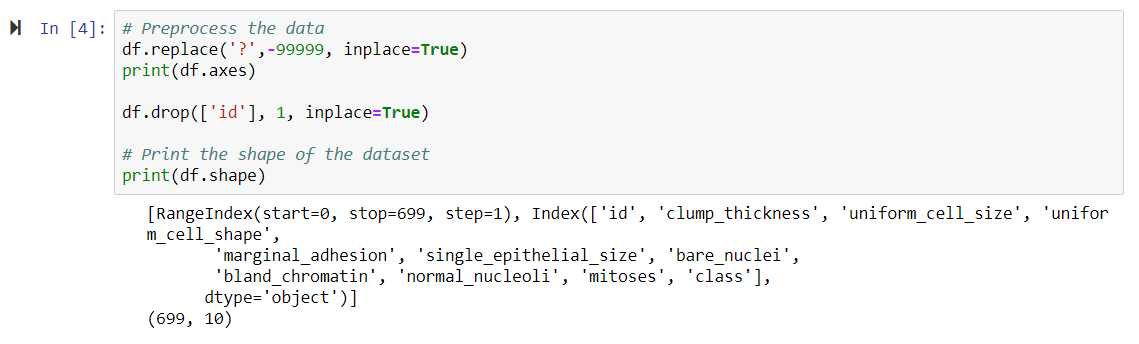As we now have all of the columns, we can detect whether the tumor is benign (which means it is non-cancerous) or malignant (which means it is cancerous). We now have 10 columns, as we have dropped the ID column.

In the following screenshot, we can see the first cell in our dataset, as well as its different features: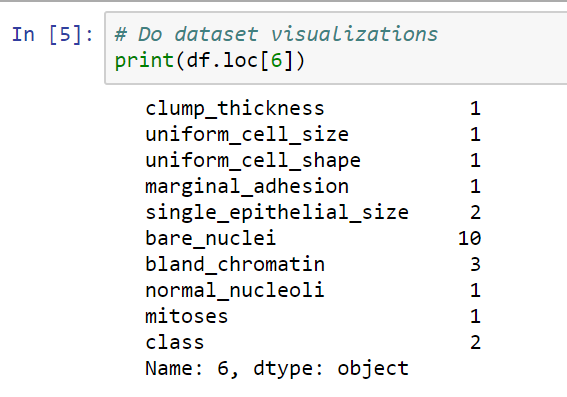Now let's visualize the parameters of the dataset, in the following steps:

1. We will print the first point, so that we can see what it entails.
2. We have a value of between 0 and 10 in all of the different columns. In the class column, the number 2 represents a benign tumor, and the number 4 represents a malignant tumor. There are 699 cells in the datasets.
3. The next step will be to do a print.describe operation, which gives us the mean, standard deviation, and other aspects for each of our different parameters or features. This is shown in the following screenshot: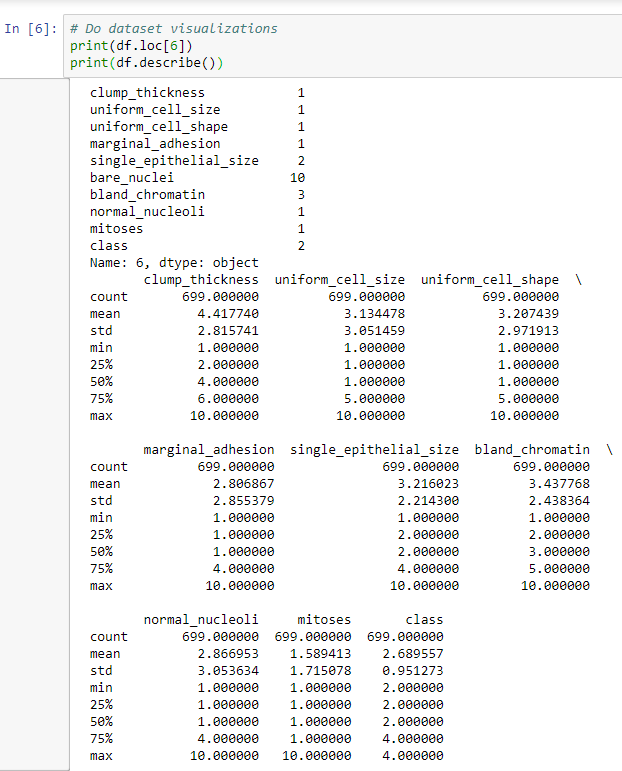Here, we have a max value of 10 for all of the different columns, apart from the class column, which will either be 2 or 4. The mean is a little closer to 2, so we have a few more benign cases than we do malignant cases. Because the min and the max values are between 1 and 10 for all columns, it means that we've successfully ignored the missing data, so we're not factoring that in. Each column has a relatively low mean, but most of them have a max of 10, which means that we have a case where we hit 10 in all but one of the classes.

# Data visualization with machine learning

Let's get started with data visualization. We will plot histograms for each variable. The steps in the preceding section are important, because we need to understand these datasets if we want to accurately and effectively use machine learning. Otherwise, we're shooting in the dark, and we might spend time on a method that doesn't need to be investigated. We will use the plt method and make a plot, in which we will add the histograms of our dataset and edit the figure sizes, to make them easier to see.

We can see the output in the following screenshot: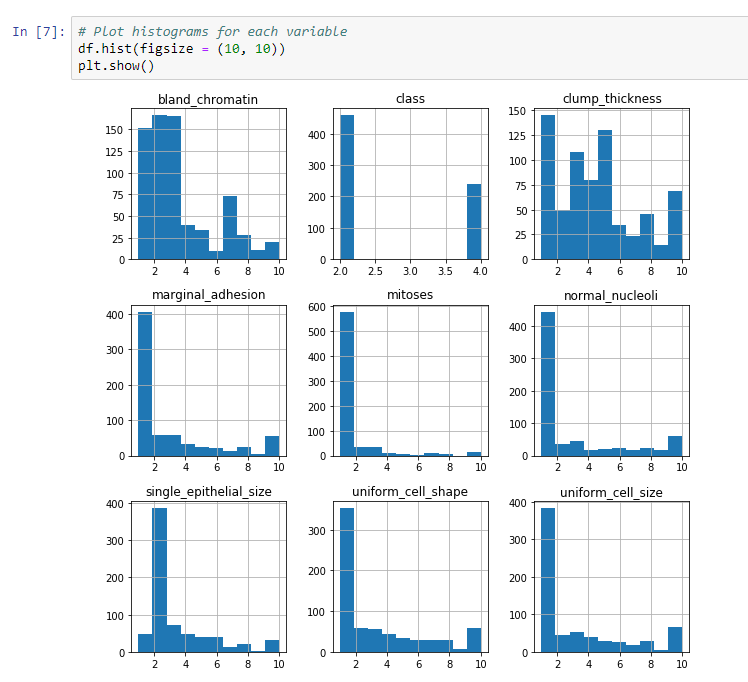As you can see, most of the preceding histograms have the majority of their data at around 1, with some data at a slightly higher value. Each histogram, apart from class, has at least one case where the value is 10. The histogram for clump thickness is pretty evenly distributed, while the histogram for chromatin is skewed to the left.

# Relationships between variables

We will now look at a scatterplot matrix, to see the relationships between some of these variables. A scatterplot matrix is a very useful function to use, because it can tell us whether a linear classifier will be a good classifier for our data, or whether we have to investigate more complicated methods.

We will add a scatter_matrix method and adjust the size to figsize(18, 18), to make it easier to see.

The output, as shown in the following screenshot, indicates the relationship between each variable and every other variable: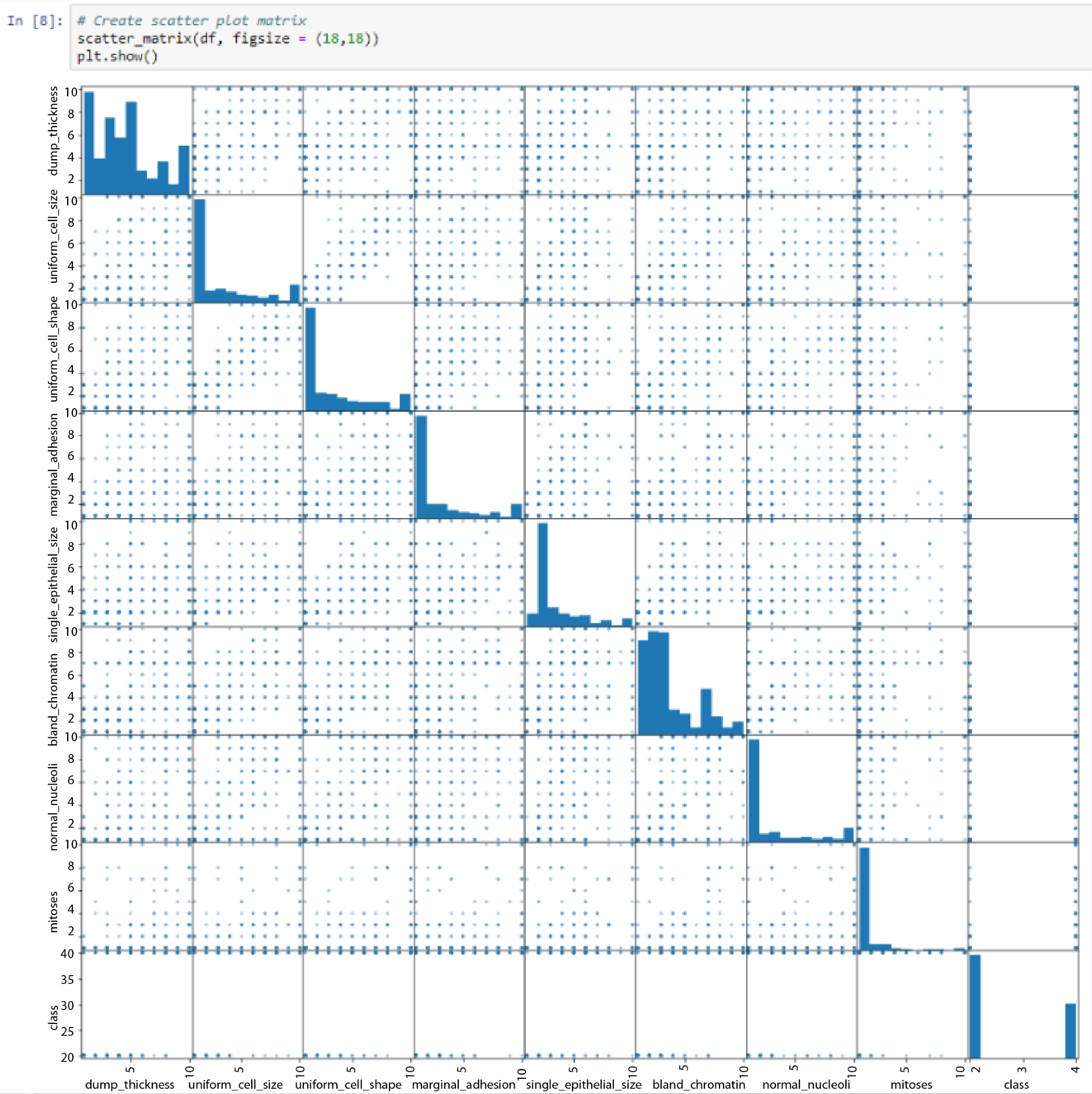All of the variables are listed on both the x and the y axes. Where they intersect, we can see the histograms that we saw previously.

In the block indicated by the mouse cursor in the preceding screenshot, we can see that there is a pretty strong linear relationship between uniform_cell_shape and uniform_cell_size. This is expected. When we go through the preceding screenshot, we can see that some other cells have a good linear relationship. If we look at our classifications, however, there's no easy way to classify these relationships.

In class in the preceding screenshot, we can see that 4 is a malignant classification. We can also see that there are cells that are scored from 1 to 10 on clump_thickness, and were still classified as malignant.

Thus, we come to the conclusion that there aren't any strong relationships between any of the variables of our dataset.

# Understanding machine learning algorithms

Since we've explored our dataset, let's take a look at how machine learning algorithms can help us to define whether a person has cancer.

The following steps will help you to better understand the machine learning algorithm:

1. The first step that we need to perform is to split our dataset into X and Y datasets for training. We won't train all of the available data, as we need to save some for our validation step. This will help us to determine how well these algorithms can generalize to new data, and not just how well they know the training data.
2. Our X data will contain all of the variables, except for the class column, and our Y data is going to be the class column, which is the classification of whether a tumor is malignant or benign.
3. Next, we will use the train_test_split function, and we will then split our data into y_train, y_test, X_train, and X_test, respectively.
1. In the same line, we will add cross_validation.train_test_split and X, y, test_size. About 20% of our data is fairly standard, so we will make the test size 0.2 to test the data as shown in the following screenshot: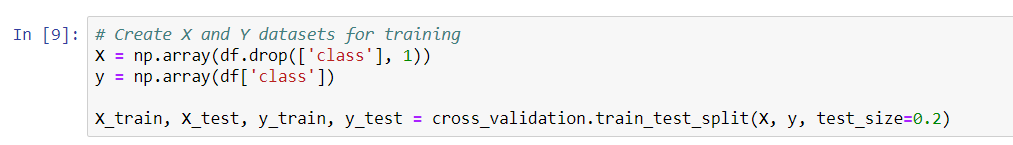1. Next, we will add a seed, which makes the data reproducible. We will start with a random seed, which will change the results a little bit every time.
If a seed is defined and we stay consistent, we should be able to reproduce our results.
1. In scoring, we will add accuracy. This is shown in the following screenshot:In the preceding section, you learned about how machine learning algorithms can be used for healthcare purposes. We also looked at the testing parameters that are used for this application.

# Training models

Now, let's move on to actually defining the training models:

1. First, make an empty list, in which we will append the KNN model.
2. Enter the KNeighborsClassifier function and explore the number of neighbors.
3. Start with n_neighbors = 5, and play around with the variable a little, to see how it changes our results.
1. Next, we will add our models: the SVM and the SVC. We will evaluate each model, in turn.
2. The next step will be to get a results list and a names list, so that we can print out some of the information at the end.
3. We will then perform a for loop for each of the models defined previously, such as name or model in models.
4. We will also do a k-fold comparison, which will run each of these a couple of times, and then take the best results. The number of splits, or n_splits, defines how many times it runs.
5. Since we don't want a random state, we will go from the seed. Now, we will get our results. We will use the model_selection function that we imported previously, and the cross_val_score.
6. For each model, we'll provide training data to X_train, and then y_train.
7. We will also add the specification scoring, which was the accuracy that we added previously.
8. We will also append results, name, and we will print out a msg. We will then substitute some variables.
9. Finally, we will look at the mean results and the standard deviation.
10. A k-fold training will take place, which means that this will be run 10 times. We will receive the average result and the average accuracy for each of them. We will use a random seed of 8, so that it is consistent across different trials and runs. Now, press Shift + Enter. We can see the output in the following screenshot: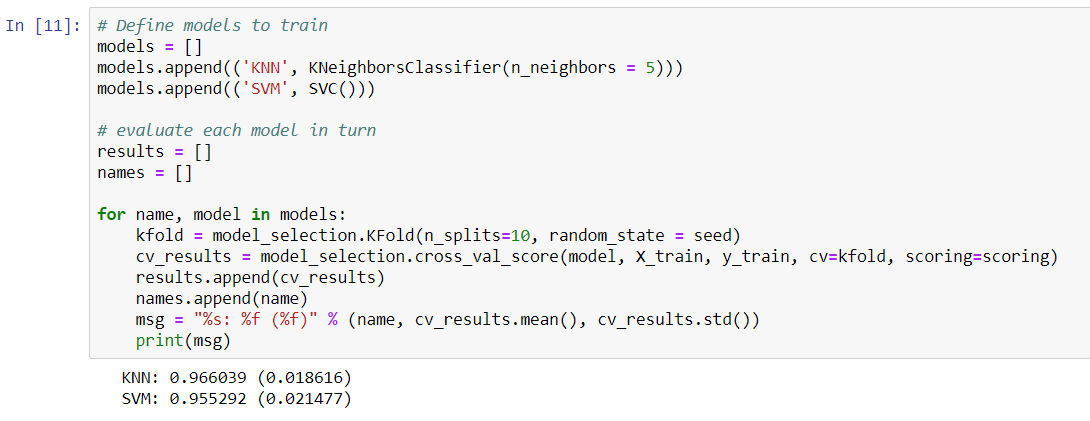In this case, our KNN narrowly beats the SVC. We will now go back and make predictions on our validation set, because the numbers shown in the preceding screenshot just represent the accuracy of our training data. If we split up the datasets differently, we'll get the following results:However, once again, it looks like we have pretty similar results, at least with regard to accuracy, on the training data between our KNN and our support vector classifier. The KNN tries to cluster the different data points into two groups: malignant and benign. The SVM, on the other hand, is looking for the optimal separating hyperplane that can separate these data points into malignant cells and benign cells.

# Predictions in machine learning

In this section, we will make predictions on the validation dataset. So far, machine learning hasn't been very helpful, because it has told us information about the training data that we already know. Let's have a look at the following steps:

1. First, we will make predictions on the validation sets with the y_test and the X_test that we split out earlier.
2. We'll do another for loop in for name, and model in models.
3. Then, we will do the model.fit, and it will train it once again on the X and y training data. Since we want to make predictions, we're going to use the model to actually make a prediction about the X_test data.
1. Once the model has been trained, we're going to use it to make a prediction. It will print out the name, the accuracy score (based on a comparison of the y_test data with the predictions we made), and a classification_report, which will tell us information about the false positives and negatives that we found.
2. Now, press Shift + Enter. The following screenshot shows the preceding steps, and the output: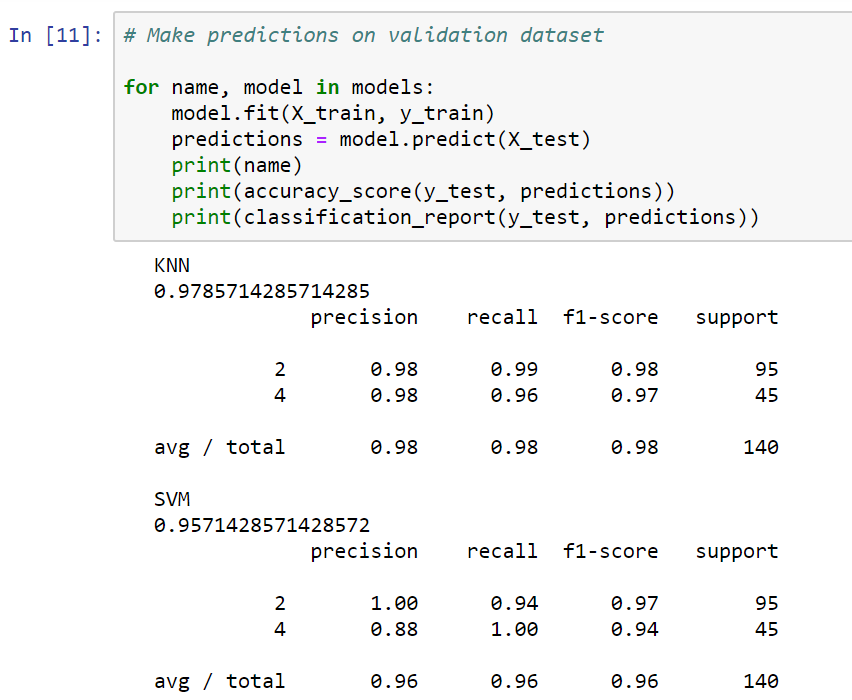In the preceding screenshot, we can see that the KNN got a 98% accuracy rating in the validation set. The SVM achieved a result that was a little higher, at 95%.

The preceding screenshot also shows some other measures, such as precision, recall, and the f1-score. The precision is a measure of false positives. It is actually the ratio of correctly predicted positive observations to the total predicted positive observations. A high value for precision means that we don't have too many false positives. The SVM has a lower precision score than the KNN, meaning that it classified a few cases as malignant when they were actually benign. It is vital to minimize the chance of getting false positives in this case, especially because we don't want to mistakenly diagnose a patient with cancer.

The recall is a measure of false negatives. In our KNN, we actually have a few malignant cells that are getting through our KNN without being labeled. The f1-score column is a combination of the precision and recall scores.

We will now go back, to do another split and randomly sort our data again. In the following screenshot, we can see that our results have changed:This time, we did much better on both the KNN and the SVM. We also got much higher precision scores from both, at 97%. This means that we probably only got one or two false positives for our KNN. We had no false negatives for our SVM, in this case.

We will now look into another example of predicting, once again based on the cell features:

1. First, we will make an SVC and get an accuracy score for it, based on our testing data.
2. Next, we will add an example. Type in np.array and pick whichever data points you want. We're going to need 10 of them. We also need to remember to see whether we get a malignant prediction.
3. We will then take example and add reshape to it. We will flip it around, so that we get a column vector.
4. We will then print our prediction and press Shift + Enter.

The following screenshot shows that we actually did get a malignant prediction: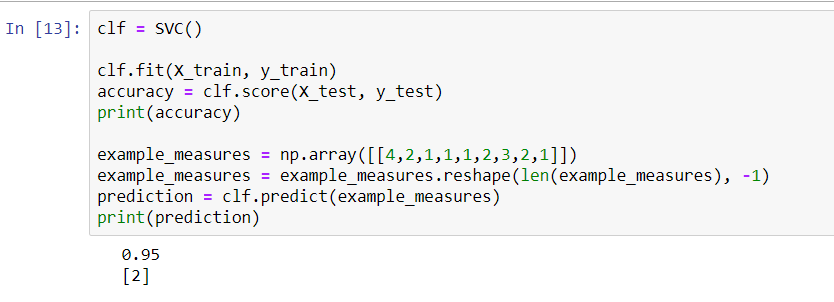In the preceding screenshot, we can see that we are 96% accurate, which is exactly what we were previously. By using the same model, we are actually able to predict whether a cell is malignant, based on its data.

When we run it again, we get the following results: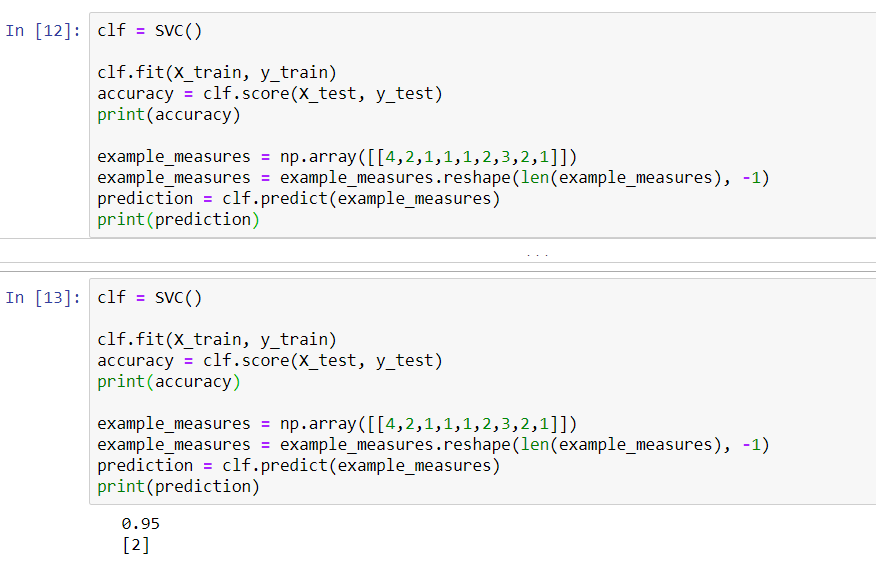By changing the example from 1 to 10, the cells go from a malignant classification to a benign classification. When we change the values in the example from 4 to 5, we learn that 4 means that it is malignant. Thus, the difference between a 4 and a 5 is enough to switch our SVM from thinking it's a malignant cell to a benign cell.

# Summary

In this chapter, we imported data from the UCI repository. We named the columns (or features), and then put them into a pandas DataFrame. We preprocessed our data and removed the ID column. We also explored the data, so that we would know more about it. We used the describe function, which gave us features such as the mean, the maximum, the minimum, and the different quartiles. We also created some histograms (so that we could understand the distributions of the different features) and a scatterplot matrix (so that we could look for linear relationships between the variables).

We then split our dataset up into a training set and a testing validation set. We implemented some testing parameters, built a KNN classifier and an SVC, and compared their results using a classification report. This consisted of features such as accuracy, overall accuracy, precision, recall, F1 score, and support. Finally, we built our own cell and explored what it would take to actually get a malignant or benign classification.

In the next chapter, you will learn about the detection of diabetes. Stay tuned for more!